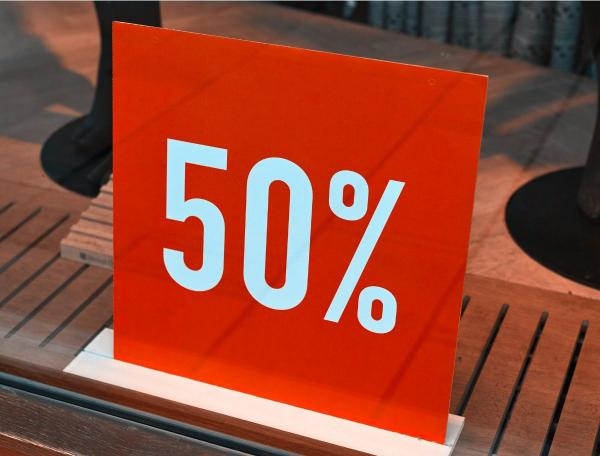# The power of equivalence

10 - 11 yearsDeveloped in partnership with Education Services Australia

The Australian Curriculum sets the goal for what all students should learn as they progress through their school life. Skills in the Year 5-6 curriculum include:

• solving problems using equivalent fractions, decimals and simple percentages through addition and subtraction
• visualising, describing and ordering equivalent fractions, decimals and simple percentages
(for example, 25% = 25 out of 100 = ¼ = 0.25)
• comparing, ordering and representing decimals up to thousandths and beyond
• finding a simple fraction or percentage of a quantity where the result is a whole number.

It’s easy to help your child practise these skills as part of everyday life – just use these simple ideas.

## Use equivalence!

Equivalence sounds technical, but we use it every day to simply calculations – eg turning 33% x \$300 into \$300 divided by three.  These ideas will help your child become familiar with these shortcuts, and master how to use them:

• when you're out shopping, talk aloud as you convert between percentages, decimals and fractions to make calculations easier
This \$40 shirt is 50% off. That means it’s half price, so we can just divide the price by two. \$40 divided by two is \$20 – what a bargain!
• ask your child to ‘remind’ you of conversion shortcuts when calculating a saving
This wheelbarrow is 33% off. It sounds like a good deal, but I can’t remember the easy way to work out the saving. Can you remind me? (Answer: 33% is the same as one third, so divide the original price by 3)
• ask your child to work out discounts, and encourage them to use conversion shortcuts
Your favourite ice cream is 25% off this week. The usual price is \$8, so how much will we save? (Shortcut: 25% is the same as one quarter, so divide \$8 by 4)
• with more difficult calculations, talk through problems to show how you estimate the answer (and check it with a calculator if needed)
These \$12 DVDs are marked down by 35%. That's just over 33%, which is one third, so the discount will be \$12 divided by three = \$4, plus a bit. Do you think that's a good deal?

## Go online

For online reinforcement, Fraction fiddle: hit the apple will give your child practice at:

• making fractions that add up to one whole.

Playground percentages will give your child practice at:

• visualising equivalent fractions and percentages on a grid of 100 squares.

[5-6Learning]

Toolkits
Tags